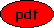On the Numerical Integration of Singular Initial and Boundary Value Problems for Generalised Lane-Emden and Thomas-Fermi Equations Co-author(s): Matthias Seiß Reference: Preprint Kassel University 2022 Description: We propose a geometric approach for the numerical integration of singular initial value problems for (systems of) quasi-linear differential equations. It transforms the original problem into the problem of computing the unstable manifold at a stationary point of an associated vector field and thus into one which can be solved in an efficient and robust manner. Using the shooting method, our approach also works well for boundary value problems. As examples, we treat some (generalised) Lane-Emden equations and the Thomas-Fermi equation. PDF File:(551 kB) Home, Last update: Thu Nov 24 18:05:35 2022